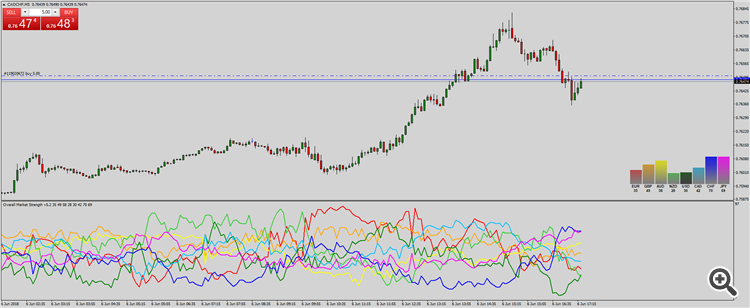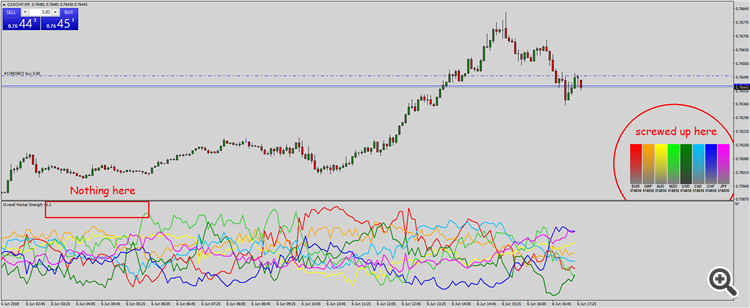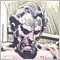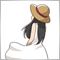# Indicator freezes on start of new bar289

So I have built my own custom currency strength meter. Here is an extract from the code

```//--- input parameters
input int      Delta=8;
extern ENUM_BASE_CORNER Corner=3;  // Panel side

//--- global variables

//--- indicator buffers
double         EURBuffer[];
double         GBPBuffer[];
double         AUDBuffer[];
double         NZDBuffer[];
double         USDBuffer[];
double         CHFBuffer[];
double         JPYBuffer[];
//+------------------------------------------------------------------+
//| Custom indicator initialization function                         |
//+------------------------------------------------------------------+
int OnInit()
{
//--- set chart properties
CreatePanel();

//--- indicator buffers mapping
IndicatorDigits(0);

SetIndexBuffer(0,EURBuffer);
SetIndexBuffer(1,GBPBuffer);
SetIndexBuffer(2,AUDBuffer);
SetIndexBuffer(3,NZDBuffer);
SetIndexBuffer(4,USDBuffer);
SetIndexBuffer(6,CHFBuffer);
SetIndexBuffer(7,JPYBuffer);

return(INIT_SUCCEEDED);
}
//+------------------------------------------------------------------+
//| Custom indicator deinitialization function                       |
//+------------------------------------------------------------------+
int deinit()
{

ObjectsDeleteAll();

return(0);
}
//+------------------------------------------------------------------+
//| Custom indicator iteration function                              |
//+------------------------------------------------------------------+
int OnCalculate(const int rates_total,
const int prev_calculated,
const datetime &time[],
const double &open[],
const double &high[],
const double &low[],
const double &close[],
const long &tick_volume[],
const long &volume[],
{
int start_index=prev_calculated;

if(prev_calculated>0)  start_index--;

for(int i=start_index;i<rates_total;i++)
{
double egopen  = iOpen("EURGBP",0,i+Delta-1);
double egclose = iClose("EURGBP",0,i);
double eghigh  = iHigh("EURGBP",0,(iHighest("EURGBP",0,MODE_HIGH,Delta,i)));
double eglow   = iLow("EURGBP",0,(iLowest("EURGBP",0,MODE_LOW,Delta,i)));
double egbody  = MathAbs(egclose-egopen);
double egwick  = MathAbs(eghigh-eglow);
double eg      = 50;

if(egbody>=Point()*10  && egwick>=Point()*20)
{
if(egclose>egopen) eg = 50+((egbody*100/egwick)/2);
else eg = 50-((egbody*100/egwick)/2);
}
//--- code repeats for each of the 28 main pairs
//--- code repeats for each of the 28 main pairs
//--- code repeats for each of the 28 main pairs

EURBuffer[i] = (eg+ea+en+eu+ec+eh+ej)/7;
GBPBuffer[i] = ((100-eg)+ga+gn+gu+gc+gh+gj)/7;
AUDBuffer[i] = ((100-ea)+(100-ga)+an+au+ac+ah+aj)/7;
NZDBuffer[i] = ((100-en)+(100-gn)+(100-an)+nu+nc+nh+nj)/7;
USDBuffer[i] = ((100-eu)+(100-gu)+(100-au)+(100-nu)+uc+uh+uj)/7;
CHFBuffer[i] = ((100-eh)+(100-gh)+(100-ah)+(100-ah)+(100-uh)+(100-ch)+cj)/7;
JPYBuffer[i] = ((100-ej)+(100-gj)+(100-aj)+(100-aj)+(100-uj)+(100-cj)+(100-hj))/7;

UpdateBarGraph();
}

return(rates_total);
}
//+------------------------------------------------------------------+

//+------------------------------------------------------------------+
//|  Update Bar Graph                                                |
//+------------------------------------------------------------------+
void UpdateBarGraph()
//+------------------------------------------------------------------+
{
objectSetBlank("label_001");
objectSetGraph("label_001_",EURBuffer,C'128,128,128',C'134,121,121',C'140,115,115',C'147,108,108',C'153,102,102',C'159,96,96',C'166,89,89',C'172,83,83',C'179,77,77',C'185,70,70',C'191,64,64',C'198,57,57',C'204,51,51',C'210,45,45',C'217,38,38',C'223,32,32',C'230,25,25',C'236,19,19',C'242,13,13',C'249,6,6',C'255,0,0');
ObjectSetString(0,"label_017",OBJPROP_TEXT,DoubleToString(EURBuffer,0));

objectSetBlank("label_002");
objectSetGraph("label_002_",GBPBuffer,C'128,128,128',C'134,129,121',C'140,131,115',C'147,133,108',C'153,135,102',C'159,137,96',C'166,139,89',C'172,141,83',C'179,143,77',C'185,145,70',C'191,147,64',C'198,149,57',C'204,150,51',C'210,152,45',C'217,154,38',C'223,156,32',C'230,158,25',C'236,160,19',C'242,162,13',C'249,164,6',C'255,165,0');
ObjectSetString(0,"label_018",OBJPROP_TEXT,DoubleToString(GBPBuffer,0));

objectSetBlank("label_003");
objectSetGraph("label_003_",AUDBuffer,C'128,128,128',C'134,134,121',C'140,140,115',C'147,147,108',C'153,153,102',C'159,159,96',C'166,166,89',C'172,172,83',C'179,179,77',C'185,185,70',C'191,191,64',C'198,198,57',C'204,204,51',C'210,210,45',C'217,217,38',C'223,223,32',C'230,230,25',C'236,236,19',C'242,242,13',C'249,249,6',C'255,255,0');
ObjectSetString(0,"label_019",OBJPROP_TEXT,DoubleToString(AUDBuffer,0));

objectSetBlank("label_004");
objectSetGraph("label_004_",NZDBuffer,C'128,128,128',C'121,134,121',C'115,140,115',C'108,147,108',C'102,153,102',C'96,159,96',C'89,166,89',C'83,172,83',C'77,179,77',C'70,185,70',C'64,191,64',C'57,198,57',C'51,204,51',C'45,210,45',C'38,217,38',C'32,223,32',C'25,230,25',C'19,236,19',C'13,242,13',C'6,249,6',C'0,255,0');
ObjectSetString(0,"label_020",OBJPROP_TEXT,DoubleToString(NZDBuffer,0));

objectSetBlank("label_005");
objectSetGraph("label_005_",USDBuffer,C'64, 64, 64',C'61, 67, 61',C'57, 70, 57',C'54, 73, 54',C'51, 77, 51',C'48, 80, 48',C'45, 83, 45',C'41, 86, 41',C'38, 89, 38',C'35, 92, 35',C'32, 96, 32',C'29, 99, 29',C'25, 102, 25',C'22, 105, 22',C'19, 108, 19',C'19, 108, 19',C'13, 115, 13',C'10, 118, 10',C'6, 121, 6',C'3, 124, 3',C'0, 128, 0');
ObjectSetString(0,"label_021",OBJPROP_TEXT,DoubleToString(USDBuffer,0));

objectSetBlank("label_006");
objectSetGraph("label_006_",CADBuffer,C'128, 128, 128',C'121, 131, 134',C'115, 134, 140',C'108, 137, 147',C'102, 140, 153',C'96, 143, 159',C'89, 147, 166',C'83, 150, 172',C'77, 153, 179',C'70, 156, 185',C'64, 159, 191',C'57, 163, 198',C'51, 166, 204',C'45, 169, 210',C'38, 172, 217',C'32, 175, 223',C'25, 179, 230',C'19, 182, 236',C'13, 185, 242',C'6, 188, 249',C'0, 191, 255');

objectSetBlank("label_007");
objectSetGraph("label_007_",CHFBuffer,C'128, 128, 128',C'121, 121, 134',C'115, 115, 140',C'108, 108, 147',C'102, 102, 153',C'96, 96, 159',C'89, 89, 166',C'83, 83, 172',C'77, 77, 179',C'70, 70, 185',C'64, 64, 191',C'57, 57, 198',C'51, 51, 204',C'45, 45, 210',C'38, 38, 217',C'32, 32, 223',C'25, 25, 230',C'19, 19, 236',C'13, 13, 242',C'6, 6, 249',C'0, 0, 255');
ObjectSetString(0,"label_023",OBJPROP_TEXT,DoubleToString(CHFBuffer,0));

objectSetBlank("label_008");
objectSetGraph("label_008_",JPYBuffer,C'128, 128, 128',C'134, 121, 134',C'140, 115, 140',C'147, 108, 147',C'153, 102, 153',C'159, 96, 159',C'166, 89, 166',C'172, 83, 172',C'179, 77, 179',C'185, 70, 185',C'191, 64, 191',C'198, 57, 198',C'204, 51, 204',C'210, 45, 210',C'217, 38, 217',C'223, 32, 223',C'230, 25, 230',C'236, 19, 236',C'242, 13, 242',C'249, 6, 249',C'255, 0, 255');
ObjectSetString(0,"label_024",OBJPROP_TEXT,DoubleToString(JPYBuffer,0));
}
//+------------------------------------------------------------------+```

Looks like this when first applied to a charthowever as soon as a new bar is formed the indicator freezes leaving me with thisCan you help the worlds dumbest programmer. Thanks in advance

P.S I am happy to attach the full code if needed. It is just however the graphics component of the indicator.3272

Robert Browne:

however as soon as a new bar is formed the indicator freezes leaving me with this

Any error message(s) in the Experts tab?289

Anthony Garot:
Any error message(s) in the Experts tab?

None whatsoever3272

Robert Browne:

None whatsoever

Well, I don't see anything immediately obvious that would cause it to freeze without an error message.

Double check that objectSetBlank() and objectSetGraph() aren't creating new objects every time. MT5 really starts to bog down on me when I have a few hundred thousands graphical objects on a chart.

Also . . . try moving the call to UpdateBarGraph() out of OnCalculate(). Updating every second in OnTimer() might be enough to be useful, and maybe that's where the freeze happens.289

Anthony Garot:

Updating every second in OnTimer() might be enough to be useful, and maybe that's where the freeze happens.

Will do that as it's what I do on other version.

But to me, it doesn't explain why I no longer get the buffer values???525

Is this an mq5 file?

MT4 cannot calculate with this method.

```int start_index=prev_calculated;

if(prev_calculated>0)  start_index--;

for(int i=start_index;i<rates_total;i++)
{
```289

Is this an mq5 file?

MT4 cannot calculate with this method.

It is mq4525

```int limit;

if (prev_calculated == 0)
limit = rates_total - Delta;
else
limit = rates_total - prev_calculated + 1;

for (int i = limit; i >= 0; i--)
{
```289

I'll give it a whirl. Thanks for the suggestion. Will get back and let you know how it goes11

The loop cannot terminate by itself, I guess289

Indicator freezes on start of new bar

```int limit;

if (prev_calculated == 0)
limit = rates_total - Delta;
else
limit = rates_total - prev_calculated + 1;

for (int i = limit; i >= 0; i--)
{
```

So I tried the code and it didn't work. But it got me thinking and I did this instead

```int limit;

if (prev_calculated == 0)
limit = rates_total - Delta;
else
limit = rates_total - prev_calculated + 1;

for(int i=0; i<limit; i++)```

And for now it appears problem solved.

Which then leads to the next question. Why does it now work? Whats the logic the program is now applying that means one code doesn't work yet the smallest of variations and then it does.